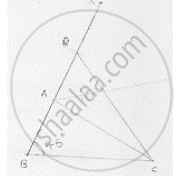# Construct a δAbc in Which Ab + Ac = 5.6 Cm, Bc = 4.5 Cm, Ab − Ac = 1.5 Cm and ∠B = 45°. - Mathematics

Construct a ΔABC in which AB + AC = 5.6 cm, BC = 4.5 cm, AB − AC = 1.5 cm and ∠B = 45°.

#### SolutionSteps of construction:
Step 1: Draw a line segment BC of 4.5cm
Step 2: At B, draw an angle XBC of 45°
Step 3: With center B and radius 5 6 , cm  draw an arc which intersects BX at D.
Step 4: Join DC
Step 5: Draw the perpendicular bisector of DC which intersects BD at A.
Step 6: Join AC
∴ΔABC is a required triangle

Concept: Some Constructions of Triangles
Is there an error in this question or solution?

#### APPEARS IN

RD Sharma Mathematics for Class 9
Chapter 16 Constructions
Exercise 16.3 | Q 2 | Page 18

Share